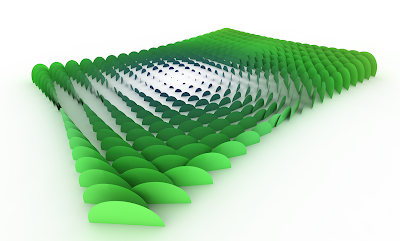## 07 September 2011

### rh_Object scale, shade color and material from an attractor pointI wrote these scripts for testing the use of distance value from a group of object to one attractor to define the scale of objects themselves, color shade and material color. It's just for fun and for exercise with VBrhinoscript.

D

Option Explicit
'this script plays with an attractor point
'Script written by davide del giudice
MsgBox "Code by Davide del Giudice www.Co-de-iT.com"
Call Main()
Sub Main()

Dim arrpts, arrpt,arrNormal
Dim i,u
Dim dbdist,strline1,arrPlane,circle
'select a grid of points
arrpts = Rhino.GetPointCoordinates ("Select grid of points",1)
'select an attractor
arrpt= Rhino.GetPointCoordinates ("Select attractor")
If IsNull (arrpts) Then
Exit Sub
If IsNull (point) Then
Exit Sub
End If
End If
'loop for the grid of points
For i= 0 To Ubound (arrpts)
'loop for the attractor
For u = 0 To UBound (arrpt)
'calculates the distance between every points to the attractor
dbdist = Rhino.Distance (arrpts(i), arrpt(u))
'draws one line from i-point and the attractor
'defines the shade color of the line
Rhino.ObjectColor strline1, rgb (dbdist*6,dbdist*15,100)
'calculates a vector from i-point and the attractor
arrNormal = Rhino.VectorCreate (arrpts (i), arrpt(0))
arrPlane=Rhino.PlaneFromNormal(arrpts(i), arrNormal)
Dim planarsrf
Rhino.ObjectColor planarsrf, rgb (dbdist*6,dbdist*15,100)
'defines the material of the planar surface
Dim material
Call rhino.MaterialColor (material, rgb (dbdist*6,dbdist*15,100))
Call rhino.MaterialShine (material, 255)

Next
Next

Option Explicit
MsgBox "Code by Davide del Giudice www.Co-de-iT.com"
Call ArrayPointsOnSurface()
Sub ArrayPointsOnSurface()

Dim strObject, nRows, nColumns,strpt,oript,arrplane,arrplane2, strLoftSRF
Dim U, V, i, j, arrParam(1), arrPoint,endpt,pts,arrClosest,arrNormal,Line,d2,pt,plane,circle,circle2

' Get the surface object
strObject = Rhino.GetObject("Select surface", 8)
oript= rhino.GetObject("pickpoint",1)
strpt= rhino.pointcoordinates(oript)

If IsNull(strObject) Then Exit Sub

' Get the number of rows
nRows = Rhino.GetInteger("Number of rows", 20, 2)
If IsNull(nRows) Then Exit Sub

nRows = nRows - 1
' Get the number of columns
nColumns = Rhino.GetInteger("Number of columns", 20, 2)

If IsNull(nColumns) Then Exit Sub

nColumns = nColumns - 1

' Get the domain of the surface
U = Rhino.SurfaceDomain(strObject, 0)
V = Rhino.SurfaceDomain(strObject, 1)
If Not IsArray(U) Or Not IsArray(V) Then Exit Sub

For i = 0 To nRows
arrParam(0) = U(0) + (((U(1) - U(0)) / nRows) * i)
For j = 0 To nColumns
arrParam(1) = V(0) + (((V(1) - V(0)) / nColumns) * j)
arrPoint = Rhino.EvaluateSurface(strObject, arrParam)

If IsArray(arrPoint) Then
arrClosest = Rhino.SurfaceClosestPoint(strObject, arrPoint)
arrNormal = Rhino.SurfaceNormal(strObject, arrParam)

Rhino.Print Rhino.Pt2Str(arrNormal)
d2=rhino.Distance (arrpoint,strpt)
arrPlane= Rhino.PlaneFromNormal (arrPoint, arrNormal)
arrPlane2= Rhino.PlaneFromNormal (endpt, arrNormal)
Dim material